# 基于改进Heston模型的欧式期权定价European Option Pricing Based on Improved Heston Model

• 全文下载: PDF(973KB)    PP.120-132   DOI: 10.12677/AAM.2020.91015
• 下载量: 105  浏览量: 184   科研立项经费支持

Heston模型是一种被广泛应用于资产管理的随机波动率(SV)模型。本文设置动态的均值回复幂函数代替Heston模型采用均值回复平方根描述波动率过程，提出一种改进的Heston模型，并根据实际确定幂函数具体形式。利用∆-对冲原理构造无风险资产组合，得到该模型下期权价格满足的PDE，以及利用反演定理求期权价格的封闭形式解。实证分析表明，改进的Heston模型能有效提高模型定价的准确性。

Heston model is a stochastic volatility (SV) model widely used in asset management. In this paper, a dynamic mean reversion power function is set to replace the Heston model which used the mean reversion square root to describe the volatility process. An improved Heston model is proposed and the specific form of power function is determined according to the actual situation. Using the ∆-hedging principle, we construct a risk-free asset portfolio, get the PDE of option price satisfying the model, and use the inversion theorem to find the closed form solution of option price. Empirical analysis shows that the improved Heston model can effectively improve the accuracy of pricing.

1. 引言

Heston模型给出欧式期权定价封闭形式的解，有效提高参数估计方面的效力。但由于模型采用固定的均值回复平方根描述波动率过程，导致有时模型计算结果与实际有较大的误差。为了克服Heston模型的不足，本文设置动态的均值回复幂函数描述波动率过程，并根据具体的实际情况确定幂函数的具体形式，提高模型的适应性和计算精度。

2. Heston模型的改进

SV模型主要基于资产价格和波动率都遵循“有偏的随机游走”，即一个漂移项加上一个随机项 ，且随机项服从标准的布朗运动。以下是考虑资产及其方差波动率都是随机过程的Stein-stein模型与Heston。

2.1. Stein-Stein  模型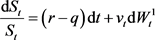$\text{d}{v}_{t}=k\left(\xi -{v}_{t}\right)\text{d}t+\sigma \text{d}{W}_{t}^{2}$

SV模型中含dt的项均为漂移项，含 $\text{d}{W}_{t}$ 项为随机项，例如，stein-stein模型中瞬时波动率的漂移项为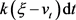，随机项为 $\sigma \text{d}{W}_{t}^{2}$，反映了波动率在该时刻先进行一个固定漂移再进行一定的随机变动。

2.2. Heston  模型

Heston  改进了stein-stein模型的随机项，提出如下随机波动率模型

$\frac{\text{d}{S}_{t}}{{S}_{t}}=\left(r-q\right)\text{d}t+\sqrt{{v}_{t}}\text{d}{W}_{t}^{1}$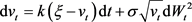2.3. 改进的Heston模型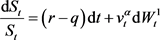$\text{d}{v}_{t}=k\left(\xi -{v}_{t}\right)\text{d}t+\sigma {v}_{t}^{\beta }\text{d}{W}_{t}^{2}$ (2-1)

3. 改进Heston模型下欧式看涨期权的PDE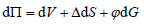(3-1)

$\text{d}G=\frac{\partial G}{\partial t}\text{d}t+\frac{\partial G}{\partial S}\text{d}S+\frac{\partial G}{\partial v}\text{d}v+\frac{1}{2}{v}^{2\alpha }{S}^{2}\frac{{\partial }^{2}G}{\partial {S}^{2}}\text{d}t+\sigma {v}^{\alpha +\beta }\rho S\frac{{\partial }^{2}G}{\partial S\partial v}\text{d}t+\frac{1}{2}{\sigma }^{2}{v}^{2\beta }\frac{{\partial }^{2}G}{\partial {v}^{2}}\text{d}t$ (3-2)

$\text{d}V=\frac{\partial V}{\partial t}\text{d}t+\frac{\partial V}{\partial S}\text{d}S+\frac{\partial V}{\partial v}\text{d}v+\frac{1}{2}{v}^{2\alpha }{S}^{2}\frac{{\partial }^{2}V}{\partial {S}^{2}}\text{d}t+\sigma {v}^{\alpha +\beta }\rho S\frac{{\partial }^{2}V}{\partial S\partial v}\text{d}t+\frac{1}{2}{\sigma }^{2}{v}^{2\beta }\frac{{\partial }^{2}V}{\partial {v}^{2}}\text{d}t$ (3-3)

$\begin{array}{c}\text{d}\Pi =\left[\frac{\partial V}{\partial t}+\frac{1}{2}{v}^{2\alpha }{S}^{2}\frac{{\partial }^{2}V}{\partial {S}^{2}}+\sigma {v}^{\alpha +\beta }\rho S\frac{{\partial }^{2}V}{\partial S\partial v}+\frac{1}{2}{\sigma }^{2}{v}^{2\beta }\frac{{\partial }^{2}V}{\partial {v}^{2}}\right]\text{d}t\\ \text{\hspace{0.17em}}\text{\hspace{0.17em}}+\phi \left[\frac{\partial G}{\partial t}+\frac{1}{2}{v}^{2\alpha }{S}^{2}\frac{{\partial }^{2}G}{\partial {S}^{2}}+\sigma {v}^{\alpha +\beta }\rho S\frac{{\partial }^{2}G}{\partial S\partial v}+\frac{1}{2}{\sigma }^{2}{v}^{2\beta }\frac{{\partial }^{2}G}{\partial {v}^{2}}\right]\text{d}t\\ \text{\hspace{0.17em}}\text{\hspace{0.17em}}+\left[\frac{\partial V}{\partial S}+\Delta +\phi \frac{\partial G}{\partial S}\right]\text{d}S+\left[\frac{\partial V}{\partial v}+\phi \frac{\partial G}{\partial v}\right]\text{d}v\end{array}$ (3-4)

$\left\{\begin{array}{l}\frac{\partial V}{\partial S}+\Delta +\phi \frac{\partial G}{\partial S}=0\\ \frac{\partial V}{\partial v}+\phi \frac{\partial G}{\partial v}=0\end{array}$(3-5)

$\begin{array}{l}\left[\frac{\partial V}{\partial t}+\frac{1}{2}{v}^{2\alpha }{S}^{2}\frac{{\partial }^{2}V}{\partial {S}^{2}}+\sigma {v}^{\alpha +\beta }\rho S\frac{{\partial }^{2}V}{\partial S\partial v}+\frac{1}{2}{\sigma }^{2}{v}^{2\beta }\frac{{\partial }^{2}V}{\partial {v}^{2}}\right]\\ \text{ }+\phi \left[\frac{\partial G}{\partial t}+\frac{1}{2}{v}^{2\alpha }{S}^{2}\frac{{\partial }^{2}G}{\partial {S}^{2}}+\sigma {v}^{\alpha +\beta }\rho S\frac{{\partial }^{2}G}{\partial S\partial v}+\frac{1}{2}{\sigma }^{2}{v}^{2\beta }\frac{{\partial }^{2}G}{\partial {v}^{2}}\right]\text{d}t\\ =r\left(V+\Delta S+\phi G\right)\text{d}t\end{array}$ (3-6)

$\begin{array}{l}\frac{\left[\frac{\partial V}{\partial t}+\frac{1}{2}{v}^{2\alpha }{S}^{2}\frac{{\partial }^{2}V}{\partial {S}^{2}}+\sigma {v}^{\alpha +\beta }\rho S\frac{{\partial }^{2}V}{\partial S\partial v}+\frac{1}{2}{\sigma }^{2}{v}^{2\beta }\frac{{\partial }^{2}V}{\partial {v}^{2}}\right]-rV+rS\frac{\partial V}{\partial S}}{\frac{\partial V}{\partial v}}\\ =\frac{\left[\frac{\partial G}{\partial t}+\frac{1}{2}{v}^{2\alpha }{S}^{2}\frac{{\partial }^{2}G}{\partial {S}^{2}}+\sigma {v}^{\alpha +\beta }\rho S\frac{{\partial }^{2}G}{\partial S\partial v}+\frac{1}{2}{\sigma }^{2}{v}^{2\beta }\frac{{\partial }^{2}G}{\partial {v}^{2}}-rG+rS\frac{\partial G}{\partial S}\right]}{\frac{\partial G}{\partial v}}\end{array}$ (3-7)满足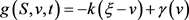$g\left(S,v,t\right)=-k\xi +\left(k+\gamma \right)v$

$g\left(S,v,t\right)$ 为关于v的线性函数，为了更加简洁，记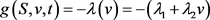，其中 ${\lambda }_{1}=k\xi$${\lambda }_{2}=-\left(k+\gamma \right)$ 将其代入(3-8)整理化简得到改进Heston模型下期权价格的PDE为

$\frac{1}{2}{v}^{2\alpha }{S}^{2}\frac{{\partial }^{2}G}{\partial {S}^{2}}+\sigma {v}^{\alpha +\beta }\rho S\frac{{\partial }^{2}G}{\partial S\partial v}+\frac{1}{2}{\sigma }^{2}{v}^{2\beta }\frac{{\partial }^{2}G}{\partial {v}^{2}}+rS\frac{\partial G}{\partial S}+\lambda \left(v\right)\frac{\partial G}{\partial v}-rG+\frac{\partial G}{\partial t}=0$ (3-9)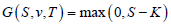$x=\mathrm{ln}S$，代入(3-9)整理化简得

$\frac{1}{2}{v}^{2\alpha }\frac{{\partial }^{2}G}{\partial {x}^{2}}-\frac{1}{2}{v}^{2\alpha }\frac{\partial G}{\partial x}+\sigma {v}^{\alpha +\beta }\rho \frac{{\partial }^{2}G}{\partial x\partial v}+\frac{1}{2}{\sigma }^{2}{v}^{2\beta }\frac{{\partial }^{2}G}{\partial {v}^{2}}+r\frac{\partial G}{\partial x}+\lambda \left(v\right)\frac{\partial G}{\partial v}-rG+\frac{\partial G}{\partial t}=0$ (3-10)

4. 改进Heston模型下欧式看涨期权定价公式

$C\left(K\right)={S}_{t}{P}_{1}\left(S,v,t\right)-K{\text{e}}^{-r\tau }{P}_{2}\left(S,v,t\right)$ (4-1)

${P}_{1}\left(S,v,t\right)$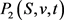分别表示欧式看涨期权按照股票价格行权的概率和在风险中性环境被行权的概率。

$C\left(K\right)={\text{e}}^{x}{P}_{1}\left(x,v,t\right)-K{\text{e}}^{-r\tau }{P}_{2}\left(x,v,t\right)$ (4-2)

(4-2)式分别求以下偏导数得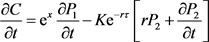$\frac{{\partial }^{2}C}{\partial {x}^{2}}={\text{e}}^{x}\left[{P}_{1}+2\frac{\partial {P}_{1}}{\partial x}+\frac{{\partial }^{2}{P}_{1}}{\partial {x}^{2}}\right]-K{\text{e}}^{-r\tau }\frac{{\partial }^{2}{P}_{2}}{\partial {x}^{2}}$

$\frac{\partial C}{\partial v}={\text{e}}^{x}\frac{\partial {P}_{1}}{\partial v}-K{\text{e}}^{-r\tau }\frac{\partial {P}_{2}}{\partial v}$(4-3)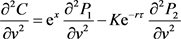$\frac{{\partial }^{2}C}{\partial x\partial v}=\left[{\text{e}}^{x}\frac{\partial {P}_{1}}{\partial v}+\frac{{\partial }^{2}{P}_{1}}{\partial x\partial v}\right]-K{\text{e}}^{-r\tau }\frac{{\partial }^{2}{P}_{2}}{\partial x\partial v}$${u}_{j}={\left(-\frac{1}{2}\right)}^{j-1}$ , ${b}_{j}=\rho \sigma \left(2-j\right)$ ,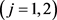，则上微分方程可化为

$\begin{array}{l}\frac{1}{2}{v}^{2\alpha }\frac{{\partial }^{2}{P}_{j}}{\partial {x}^{2}}+\sigma {v}^{\alpha +\beta }\rho \frac{{\partial }^{2}{P}_{j}}{\partial x\partial v}+\frac{1}{2}{\sigma }^{2}{v}^{2\beta }\frac{{\partial }^{2}{P}_{j}}{\partial {x}^{2}}+\left(r+{u}_{j}{v}^{2\alpha }\right)\frac{\partial {P}_{j}}{\partial x}\\ +\left(\lambda \left(v\right)+{b}_{j}{v}^{\alpha +\beta }\right)\frac{\partial {P}_{j}}{\partial v}+\frac{\partial {P}_{j}}{\partial t}=0\end{array}$ (4-4)

(4-4)是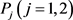满足的偏微分方程，下面通过随机变量x的特征函数给出。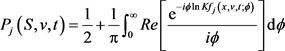(4-5)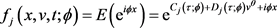(4-6)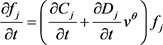$\frac{\partial {f}_{j}}{\partial x}=i\varphi {f}_{j}$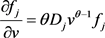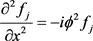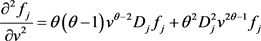$\frac{{\partial }^{2}{f}_{j}}{\partial x\partial v}=i\varphi \theta {D}_{j}{v}^{\theta -1}{f}_{j}$

$\begin{array}{l}\left[-\frac{1}{2}{\varphi }^{2}+i{u}_{j}\varphi \right]{v}^{2\alpha }+\left[i\rho {\sigma }^{2}\varphi +{b}_{j}\right]\theta {D}_{j}{v}^{\alpha +\beta +\theta -1}+\frac{1}{2}{\sigma }^{2}\theta \left(\theta -1\right){D}_{j}{v}^{2\beta +\theta -2}\\ +\frac{1}{2}{\sigma }^{2}{\theta }^{2}{D}_{j}^{2}{v}^{2\beta +2\theta -2}+\frac{\partial {D}_{j}}{\partial t}{v}^{\theta }+\left[\frac{\partial {C}_{j}}{\partial t}+ir\varphi \right]+\theta {D}_{j}\lambda \left(v\right){v}^{\theta -1}=0\end{array}$ (4-7)

1) 因为当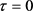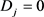，所以(4-7)可化为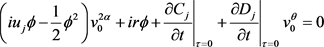(4-8)

2) 将 $\theta =2\alpha ,\lambda \left(v\right)={\lambda }_{1}+{\lambda }_{2}v$ 代入(4-8)得(4-9)

i) 若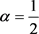，则(4-9)化为

$\begin{array}{l}\left[-\frac{1}{2}{\varphi }^{2}+i{u}_{j}\varphi +\frac{\partial {D}_{j}}{\partial t}+{D}_{j}{\lambda }_{2}\right]v+\left[i\rho {\sigma }^{2}\varphi +{b}_{j}\right]{D}_{j}{v}^{\beta +\frac{1}{2}}\\ \text{ }+\frac{1}{2}{\sigma }^{2}{D}_{j}^{2}{v}^{2\beta }+\left[\frac{\partial {C}_{j}}{\partial t}+ir\varphi +{D}_{j}{\lambda }_{1}\right]=0\end{array}$ (4-10)

$\left[i\rho {\sigma }^{2}\varphi +{b}_{j}\right]{D}_{j}{v}^{\beta +\frac{1}{2}}+\frac{1}{2}{\sigma }^{2}{D}_{j}^{2}{v}^{2\beta }=0$

ii) 若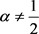。假设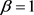，则(4-9)化为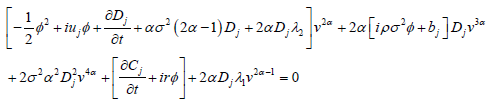(4-11)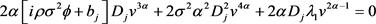$2\beta +4\alpha -2$$2\alpha -1$ 均不等于0且不等于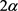。由于(4-9)对任意v恒成立，故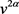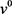的系数都为零，(4-9)可化为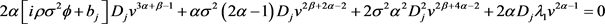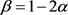，将其代入(4-9)得

$\begin{array}{l}\left[-\frac{1}{2}{\varphi }^{2}+i{u}_{j}\varphi +\frac{\partial {D}_{j}}{\partial t}+2\alpha {D}_{j}{\lambda }_{2}\right]{v}^{2\alpha }+2\alpha \left[i\rho {\sigma }^{2}\varphi +{b}_{j}\right]{D}_{j}{v}^{\alpha }+\alpha {\sigma }^{2}\left(2\alpha -1\right){D}_{j}{v}^{-2\alpha }\\ \text{ }+2{\sigma }^{2}{\alpha }^{2}{D}_{j}^{2}+\left[\frac{\partial {C}_{j}}{\partial t}+ir\varphi \right]+2\alpha {D}_{j}{\lambda }_{1}{v}^{2\alpha -1}=0\end{array}$ (4-12)

$2\alpha \left[i\rho {\sigma }^{2}\varphi +{b}_{j}\right]{D}_{j}{v}^{\alpha }+\alpha {\sigma }^{2}\left(2\alpha -1\right){D}_{j}{v}^{-2\alpha }+2\alpha {D}_{j}{\lambda }_{1}{v}^{2\alpha -1}=0$ (4-13)

$\alpha =\frac{1}{4}$ 时，(4-13)可化为

$\frac{1}{2}\left[i\rho {\sigma }^{2}\varphi +{b}_{j}\right]{D}_{j}{v}^{\frac{1}{4}}+\frac{1}{8}{D}_{j}\left(2{\lambda }_{1}-{\sigma }^{2}\right){v}^{-\frac{1}{2}}=0$ (4-14)

$\alpha \ne \frac{1}{4}$ 时，由于(4-13)对任意v恒成立，故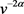的系数零，(4-13)化为

$2\alpha \left[i\rho {\sigma }^{2}\varphi +{b}_{j}+{\lambda }_{1}{v}^{\alpha -1}\right]{D}_{j}=0$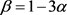，将其代入(4-9)得

$\begin{array}{l}\left[-\frac{1}{2}{\varphi }^{2}+i{u}_{j}\varphi +\frac{\partial {D}_{j}}{\partial t}+2\alpha {D}_{j}{\lambda }_{2}\right]{v}^{2\alpha }+2\alpha \left[i\rho {\sigma }^{2}\varphi +{b}_{j}\right]{D}_{j}+\alpha {\sigma }^{2}\left(2\alpha -1\right){D}_{j}{v}^{-4\alpha }\\ \text{ }+2{\sigma }^{2}{\alpha }^{2}{D}_{j}^{2}{v}^{-2\alpha }+\left[\frac{\partial {C}_{j}}{\partial t}+ir\varphi \right]+2\alpha {D}_{j}{\lambda }_{1}{v}^{2\alpha -1}=0\end{array}$ (4-15)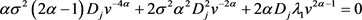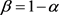代入模型(2-1)，(2-1)可转化为如下形式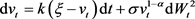(4-16)时，(4-16)即为Heston模型，欧式看涨期权定价公式采用Heston模型下欧式看涨期权定价公式。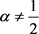时，将 $\beta =1-\alpha$ 代入(4-9)得

$\begin{array}{l}\left[-\frac{1}{2}{\varphi }^{2}+i{u}_{j}\varphi +\frac{\partial {D}_{j}}{\partial t}+2\alpha \left(i\rho {\sigma }^{2}\varphi +{b}_{j}\right){D}_{j}+2{\alpha }^{2}{\sigma }^{2}{D}_{j}^{2}+2\alpha {D}_{j}{\lambda }_{2}\right]{v}^{2\alpha }\\ +\left[\frac{\partial {C}_{j}}{\partial t}+ir\varphi +{\sigma }^{2}\alpha \left(2\alpha -1\right){D}_{j}\right]+2\alpha {D}_{j}{\lambda }_{1}{v}^{2\alpha -1}=0\end{array}$ (4-17)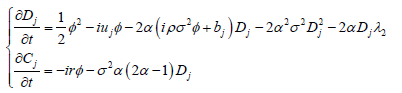(4-18)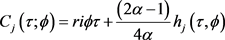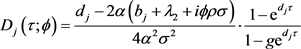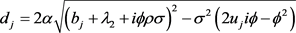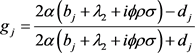(4-19)

$x=\mathrm{ln}S$

${P}_{j}=\frac{1}{2}+\frac{1}{\pi }{\int }_{0}^{\infty }Re\left[\frac{{\text{e}}^{-i\varphi \mathrm{ln}K{f}_{j}\left(x,v,t;\varphi \right)}}{i\varphi }\right]\text{d}\varphi$

${f}_{j}\left(x,v,t;\varphi \right)={\text{e}}^{{C}_{j}\left(\tau ;\varphi \right)+{D}_{j}\left(\tau ;\varphi \right){v}^{\theta }+i\varphi x}$

${C}_{j}\left(\tau ;\varphi \right)=ri\varphi \tau +\frac{2\alpha -1}{4\alpha }{h}_{j}\left(\tau ,\varphi \right)$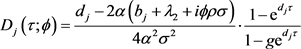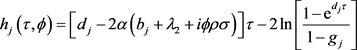${d}_{j}=2\alpha \sqrt{{\left({b}_{j}+{\lambda }_{2}+i\varphi \rho \sigma \right)}^{2}-{\sigma }^{2}\left(2{u}_{j}i\varphi -{\varphi }^{2}\right)}$

${g}_{j}=\frac{2\alpha \left({b}_{j}+{\lambda }_{2}+i\varphi \rho \sigma \right)-{d}_{j}}{2\alpha \left({b}_{j}+{\lambda }_{2}+i\varphi \rho \sigma \right)+{d}_{j}}$

${u}_{j}={\left(-\frac{1}{2}\right)}^{j-1}$

${b}_{j}=\rho \sigma \left(2-j\right)$$j=1,2$

5. 数值计算实例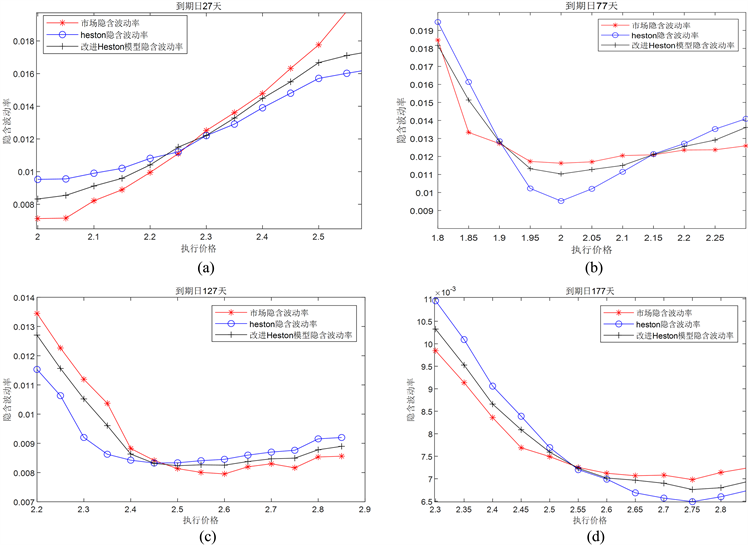Figure 1. Implied volatility chart of Shanghai 50ETF European call options

6. 结论

  Bachelier, L. (1900) Théorie de la Spéculation. Annales scientifiques de l’École Normale Supérieure, 17, 21-86. https://doi.org/10.24033/asens.476  Samuelson, P. (1965) Proof That Properly Anticipated Prices Fluctuate Randomly. Industrial Management Review, 6, 41-50.  Black, F. and Scholes, M. (1973) The Pricing of Options and Corporate Liabilities. Journal of Political Economy, 81, 637-659.  黄文礼. 基于分数布朗运动模型的金融衍生品定价[D]: [博士学位论文]. 杭州: 浙江大学, 2011.  Fama, E.F. (1965) The Behavior of Stock Market Prices. Journal of Business, 38, 34-105. https://doi.org/10.1086/294743  吴鑫育, 李心丹, 马超群. 基于随机波动率模型的上证50ETF期权定价研究[J]. 数理统计与管理, 2019, 38(1): 115-131.  Johnson, H. and Shanno, D. (1987) Option Pricing When the Variance Is Changing. Journal of Financial and Quantitative Analysis, 22, 143-151. https://doi.org/10.2307/2330709  Scott, L.O. (1987) Option Pricing When the Variance Changes Randomly: Theory, Estimation, and an Application. Journal of Financial and Quantitative Analysis, 22, 419-438. https://doi.org/10.2307/2330793  Wiggins, B. (1987) Option Values under Stochastic Volatility: Theory and Empirical Estimates. Journal of Financial Economics, 19, 351-372. https://doi.org/10.1016/0304-405X(87)90009-2  Hull, J. and White, A. (1987) The Pricing of Options on Assets with Stochastic Volatilities. The Journal of Finance, 42, 281-300. https://doi.org/10.1111/j.1540-6261.1987.tb02568.x  Stein, E.M. and Stein, J.C. (1991) Stock Price Distributions with Stochastic Volatility: An Analytical Approach. Review of Financial Studies, 4, 727-752. https://doi.org/10.1093/rfs/4.4.727  Heston, S.L. (1993) A Closed-Form Solution for Options with Stochastic Volatility with Applications to Bond and Currency Options. The Review of Financial Studies, 6, 327-343. https://doi.org/10.1093/rfs/6.2.327  Li, C.X. (2016) Bessel Processes, Stochastic Volatility, and Timer Options. Mathematical Finance, 26, 122-148. https://doi.org/10.1111/mafi.12041  马俊美, 杨宇婷, 顾桂定, 徐承龙. 随机波动率模型下基于精确模拟算法的期权计算理论[J]. 同济大学学报(自然科学版), 2017, 45(10): 1539-1548.  Hong, H., Bian, Z. and Chen, N. (2019) Leverage Effect on Stochastic Volatility for Option Pricing in Hong Kong: A Simulation and Empirical Study. The North American Journal of Economics and Finance, 2, 112-123. https://doi.org/10.1016/j.najef.2019.02.003  谢超强, 吕文元, 陈进. 基于Heston随机波动率模型和风险偏好视角的资产负债管理[J]. 运筹与管理, 2018, 27(6): 156-161.  Soleymani, F. and Barfeie, M. (2019) Pricing Options under Stochastic Volatility Jump Model: A Stable Adaptive Scheme. Applied Numerical Mathematics, 145, 69-89. https://doi.org/10.1016/j.apnum.2019.05.027  Rouah, F.D. (2013) The Heston Model and Its Extensions in Matlab and C. Wiley, Hoboken, 6-55. https://doi.org/10.1002/9781118656471  Shreve, S. (2004) Stochastic Calculus for Finance II. Springer Finance, Springer-Verlag, New York. https://doi.org/10.1007/978-1-4757-4296-1  Gil-Pelaez, J. (1951) Note on the Inversion Theorem. Biometrika, 37, 481-482. https://doi.org/10.1093/biomet/38.3-4.481